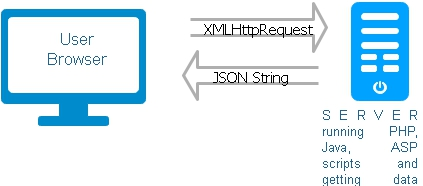# Json Data string from database recordsUser browser sends xmlhttprequest to backend server scripts to send data. We will be sending data back to the main page from web server by using Json formatted strings. These strings will contain number of Jason data value pairs taken from database table along with some other data.

Json string carries data to the parent application which we will display using JavaScript objects. To generate json string we will use php function json_encode().

We have our student table which has four fields ( id, name, class , mark). By using this we will develop some sample scripts to generate json string and then get back the data after parsing the string using JSON.parse.
Download the demo script with sample data at end of this tutorial

## Getting a single record

Let us first try by collecting and returning a single record from our student table. For better security we have used PDO to connect to MySQL data base and getting the records. We have not discussed the mysql data collection part here, only the data formatting is shown.
``````\$count=\$dbo->prepare("select id,name,class as class1,mark from student where id=:id");
\$count->bindParam(":id",\$id,PDO::PARAM_INT,5);
\$count->execute();
\$row = \$count->fetch(PDO::FETCH_OBJ);
\$main = array('data'=>array(\$row));
echo json_encode(\$main); ``````
In above code we have used json_encode function to generate the json string. Here is the output or the Json string
``{"data":[{"id":"2","name":"Max Ruin","class1":"Three5","mark":"85"}]}``
The above string is passed to JavaScript to parse the Json string and to get the data. here is the code.
``````var myObject = JSON.parse(httpxml.responseText);

var str="<table width='200'  align=center>";
for(i=0;i<myObject.data.length;i++)
{
str = str + "<tr ><td >ID:</td><td>" + myObject.data[i].id + " </td></tr>";
str = str + "<tr ><td >Name:</td><td>" + myObject.data[i].name + " </td></tr>";

str  = str + "<tr ><td>Class</td><td>"+ myObject.data[i].class1 + "</td></tr>";
str  = str + "<tr><td>Mark</td><td>"+ myObject.data[i].mark + "</td></tr>";
}

str = str + "</table>" ;

document.getElementById("display").innerHTML=str;``````
Watch the first line in above code. We have used JSON.parse to create JavaScript object. We can also use eval() like this
``var myObject = eval('(' + httpxml.responseText + ')');``
It is not a good idea to use eval() as it can execute any JavaScript script so it is a security problem. However JSON.parse is not yet supported by all the advance browsers. So if it is not supported then we can keep another external JavaScript file json2.js downloaded from https://github.com/douglascrockford/JSON-js

Along with single data row we will also try to send few more data . We are sending two arrays of data here. There are two members one is data and other one is value. Each member contains two arrays containing some objects.
``````\$main = array('data'=>array(\$row),'value'=>array("bgcolor"=>"\$bgcolor","message"=>"\$message"));
\$main = array('data'=>array(\$row));
echo json_encode(\$main); ``````
Above two lines are required changes in main code. You can see we have added message, bgcolor ( background colour ) etc. to be posted to main script. Here it is how to get the data from JavaScript object in main script.
``````\$main = array('data'=>array(\$row),'value'=>array("bgcolor"=>"\$bgcolor","message"=>"\$message"));
echo json_encode(\$main); ``````

## Array of records

We will generate json string from an array of data records. Here is the code to get data and return the Json string
``````\$sql="select *  from student where id <5";
\$row=\$dbo->prepare(\$sql);
\$row->execute();
\$result=\$row->fetchAll(PDO::FETCH_ASSOC);

\$main = array('data'=>\$result,'value'=>array("bgcolor"=>"#f1fff1","message"=>"All records displayed"));
echo json_encode(\$main);
``````
Here is the Json string as output
``````{"data":[{"id":"1","name":"John Deo","class":"Four5","mark":"75","sex":"male"},
{"id":"2","name":"Max Ruin","class":"Three5","mark":"85","sex":"male"},
{"id":"3","name":"Arnold","class":"Three5","mark":"55","sex":"male"},
{"id":"4","name":"Krish Star","class":"Four5","mark":"60","sex":"male"}],
"value":{"bgcolor":"#f1fff1","message":"All records displayed"}}``````
Using mysqli database connection
``````<?php
require "config.php"; // mysqli connection string
\$sql="SELECT name,class,mark FROM student LIMIT 0,10";
\$result = \$connection->query(\$sql);
\$row = array();
while(\$rs = \$result->fetch_array(MYSQLI_ASSOC)) {
\$row[] = \$rs;
}

echo json_encode(array("student_data"=>\$row));
\$connection->close();
?>``````

## Receiving data

In above code we have kept one array of data ( array name is data ) and some single data. Now we will learn how to receive these data and display them.
Inside your Ajax function first let us try to collect the whole bunch of data.
``var myObject = JSON.parse(httpxml.responseText);``
We know the array name is data. So we will loop through the array to display each row of record of the array.
``````var str="<table><tr><th>ID</th><th>Name</th><th>Class</th><th>Mark</th></tr>";
for(i=0;i<myObject.data.length;i++)
{
str = str + "<tr><td>" + myObject.data[i].id + " </td><td>" + myObject.data[i].name +  " </td><td>" + myObject.data[i].class + " </td><td>" + myObject.data[i].mark + "</td></tr>"
}
``````
In addition to these array of records we have some single records stored in value. Here one sample to retrieve them
``var message=myObject.value.message``
Similarly another one
``var bgcolor=myObject.value.bgcolor``

## Displaying data or calling from a file.

Different scripts can call any file which displays data in Json string. For example we can keep all the code inside one PHP file and give the name as data1.php
``````<?Php
\$my_data = new stdClass();
\$my_data->name="plus2net";
\$my_data->area="PHP";
echo json_encode(\$my_data);
?>``````
We will keep xmlhttprequest() in another file data1-read.php ( Only JavaScript code )
``````<DIV id="display"></DIV>
<script>
var httpxml = new XMLHttpRequest();

function state_check(){
//document.getElementById("display").innerHTML=httpxml.responseText;
var my_obj=JSON.parse(httpxml.responseText);
document.getElementById("display").innerHTML="NAME:" + my_obj.name+ " AREA:" + my_obj.area;
}
}
//////
httpxml.open("GET", "data1.php", true);
httpxml.send();
</script>``````

``\$.getJSON("student-data.php", function(return_data){``
We can also use Ajax to call function

To see the example on how this code is used, you can read our Ajax based PHP MySQL Paging script

Subscribe to our YouTube Channel here

## Subscribe

* indicates required
Subscribe to plus2netplus2net.com

 Minh 19-03-2015 How do you add a hyperlink onto the data that is displayed in the box?

Post your comments , suggestion , error , requirements etc here .